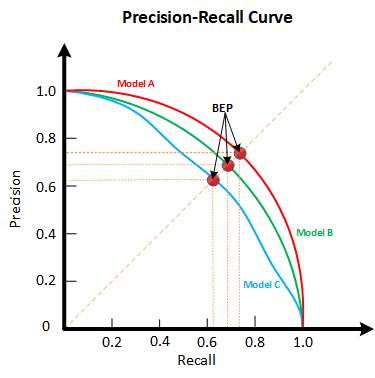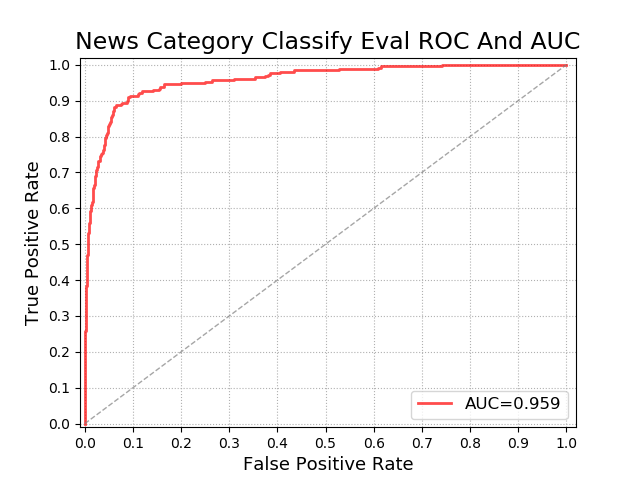# 模型评估指标（Confusion Matrix、ROC、AUC）

## 2、混淆矩阵（Confusion Matrix）

1 0

0 FP（01） TN（00）

1. TP：预测为正样本，实际也为正样本的特征数
2. FP：预测为正样本，实际为负样本的特征数
3. TN：预测为负样本，实际也为负样本的特征数
4. FN：预测为负样本，实际为正样本的特征数

### 2.1 模型整体效果：准确率

$Accuracy = \frac{TP(11)+TN(00)}{TP(11)+TN(00)+FP(01)+FN(10)}$

### 2.2 捕捉少数类：精确率（Precision）、召回率（Recall）和F1-score

#### 2.2.1 精准率(Precision)

$Precision = \frac{TP(11)}{TP(11)+FP(01)}$

#### 2.2.2 召回率(Recall)

$Recall = \frac{TP(11)}{TP(11)+FN(10)}$

#### 2.2.3 P-R曲线（Precision Recall Curve）

P-R曲线（Precision Recall Curve） 正是描述精确率/召回率变化的曲线，P-R曲线定义如下：对于同一个模型，通过调整分类阈值，可以得到不同的P-R值，从而可以得到一条曲线（纵坐标为P，横坐标为R）。通常随着分类阈值从大到小变化（大于阈值认为P），Precision减小，Recall增加。比较两个分类器好坏时，显然是查得又准又全的比较好，也就是的PR曲线越往坐标（1，1）的位置靠近越好。若一个学习器的P-R曲线被另一个学习器完全”包住”，则后者的性能优于前者。当存在交叉时，可以计算曲线围住面积，不太容易判断，但是可以通过平衡点（Break-Even Point，BEP）来判断，当P=R时的取值，平衡点的取值越高，性能更优。。如下图所示：#### 2.2.4 F1-Score

F1-Score 正如上文所述，Precision和Recall指标有时是此消彼长的，即精准率高了，召回率就下降，在一些场景下要兼顾精准率和召回率，最常见的方法就是F1-Measure，又称F1-Score。F1-Measure是P和R的加权调和平均，即：

$F1-score = \frac{2}{\frac{1}{Recall}+{\frac{1}{Precision}}} = \frac{2*Precision*Recall}{Precision+Recall}$

#### 2.2.5 sklearn中的混淆矩阵

sklearn.metrics.confusion_matrix 混淆矩阵
sklearn.metrics.accuracy_score 准确率accuracy
sklearn.metrics.precision_score 精确度precision
sklearn.metrics.recall_score 召回率recall
sklearn.metrics.precision_recall_curve 精确度-召回率平衡曲线
sklearn.metrics.f1_score F1-Score

## 3、ROC、AUC

ROC以及后面要讲到的AUC，是分类任务中非常常用的评价指标，本文将详细阐述。可能有人会有疑问，既然已经这么多评价标准，为什么还要使用ROC和AUC呢？

$TPR = \frac{正样本预测正确数}{正样本总数}= \frac{TP(11)}{TP(11)+FN(10)} = Recall$

$FPR = \frac{负样本预测错误数}{负样本总数} = \frac{FP(01)}{TN(00)+FP(01)}$

ROC曲线中的主要两个指标就是真正率TPR和假正率FPR，上面已经解释了这么选择的好处所在。其中横坐标为假正率（FPR），纵坐标为真正率（TPR），下面就是一个标准的ROC曲线图。sklearn中的ROC曲线和AUC面积

sklearn.metrics.roc_auc_score(y_true, y_score, average='macro', sample_weight=None, max_fpr=None, multi_class='raise', labels=None

sklearn.metrics.roc_curve(y_true, y_score, pos_label=None, sample_weight=None, drop_intermediate=True)


from sklearn.metrics import roc_curve
from sklearn.metrics import roc_auc_score
area = roc_auc_score(y,clf.decision_function(X))
FPR, recall, thresholds = roc_curve(y,clf.decision_function(X), pos_label=1)

plt.figure()
plt.plot(FPR, recall, color='red',label='ROC curve (area = %0.2f)' % area)
plt.plot([0, 1], [0, 1], color='black',linestyle='--')
plt.xlim([-0.05, 1.05])
plt.ylim([-0.05, 1.05])
plt.xlabel('False Positive Rate')
plt.ylabel('Recall')
plt.legend(loc="lower right")
plt.show()



#### 机器学习必学的10大算法

08-1404-18278

#### 分类模型评估——混淆矩阵、评估指标、ROC与AUC

03-073万+

#### 模型评估指标AUC（area under the curve）

06-117778

#### 【模型评估】混淆矩阵（Confusion matrix）及其指标

10-163787

#### python 画 ROC 曲线

09-111874

#### [高光谱] (6w字巨详细) GitHub开源项目Hyperspectral-Classification的解析

04-13434

#### 机器学习知识点相关总结（五）——CNN相关

11-015762

#### 形成性与反应性测量模型(Formative and Reflective Measurement Model)

08-159070

#### 机器学习模型评估混淆矩阵、ROC曲线和AUC以及PR曲线

01-251万+

#### 用tensorflow画ROC曲线©️2020 CSDN 皮肤主题: 技术工厂 设计师: CSDN官方博客点击重新获取扫码支付1.余额是钱包充值的虚拟货币，按照1:1的比例进行支付金额的抵扣。
2.余额无法直接购买下载，可以购买VIP、C币套餐、付费专栏及课程。余额充值